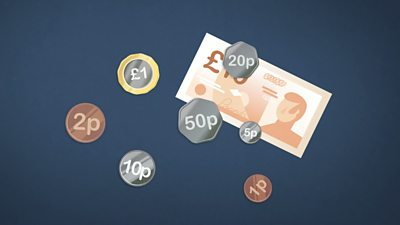# How can different coins and notes make the same total?

## Different notes and coins can make the same total

Money comes in lots of different notes and coins

Notes come in £5, £10, £20 and £50. Some Scottish banks make £100 notes too.

Coins come in 1p,2p, 5p, 10p, 20p, 50p, £1 and £2.

You can use different notes and coins to make the same amounts. The bigger the amount of money, the more ways there are to make it:

There is only 1 way to make 1p

• one 1p coin.

There are 2 ways to make 2p

• one 2p coin
• 1p + 1p

There are 4 ways to make 5p

• one 5p coin
• 2p + 2p + 1p
• 2p + 1p + 1p + 1p
• 1p + 1p + 1p+ 1p + 1p

There are 11 ways to make 10p

• one 10p coin
• 5p +5p
• 5p + 2p + 2p + 1p
• 5p + 2p + 1p + 1p + 1p
• 5p + 1p + 1p + 1p + 1p + 1p
• 2p + 2p + 2p + 2p + 2p
• 2p + 2p + 2p + 2p + 1p + 1p
• 2p + 2p + 2p + 1p + 1p + 1p + 1p
• 2p + 2p + 1p + 1p + 1p + 1p + 1p + 1p
• 2p + 1p + 1p + 1p+ 1p + 1p + 1p + 1p + 1p
• 1p + 1p + 1p + 1p + 1p + 1p + 1p + 1p + 1p + 1p

There are 41 ways to make 20p - too many to write here!

There are so many different ways of adding money that you can usually pick one that works best for you.

## There's more to learn...

This guide